# Applications of Derivatives exercise 10(f) Solutions Inter

Intermediate Mathematics 1B Applications of Derivatives exercise 10(f) textbook solutions are given.

Study the lesson Applications of Derivatives very well.

Practice the example problems and solutions given in the textbook.

Observe the given below solutions and try them in your own method

You can also see

Inter Maths 1A textbook solutions

Inter Maths 1B textbook solutions

Inter Maths IIA textbook solutions

Inter Maths IIB textbook solutions

Applications of Derivatives

Exercise 10(a)

Exercise 10(b)

Exercise 10(c)

Exercise 10(d)

Exercise 10(e)

Exercise 10(f)

Exercise 10(g)

Exercise 10(h)

M

## Exercise 10(f) Solutions Applications of Derivatives

Chapter 10 – Applications of Derivatives

Exercise 10(f)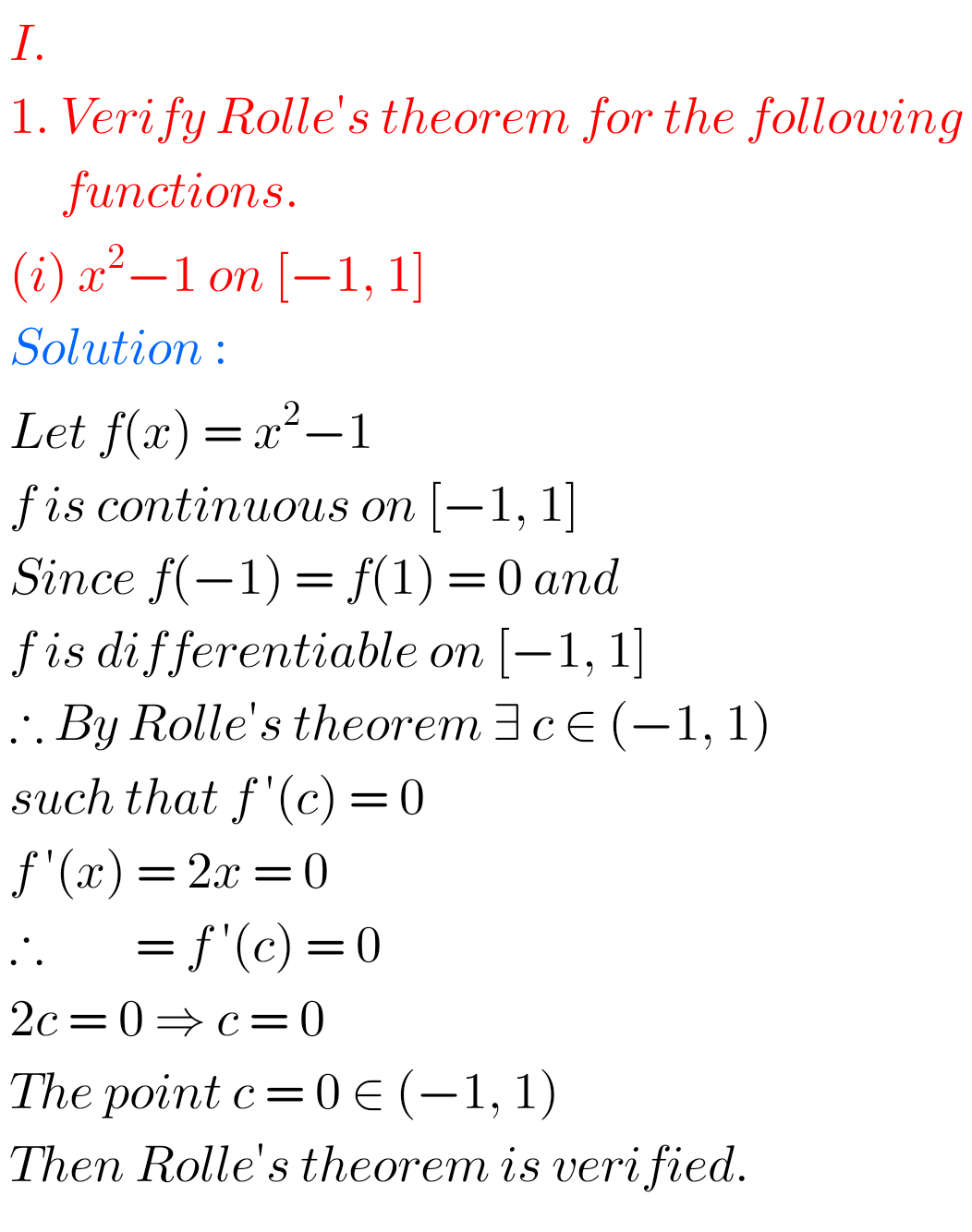## Applications of Derivatives solutions exercise 10(f) Inter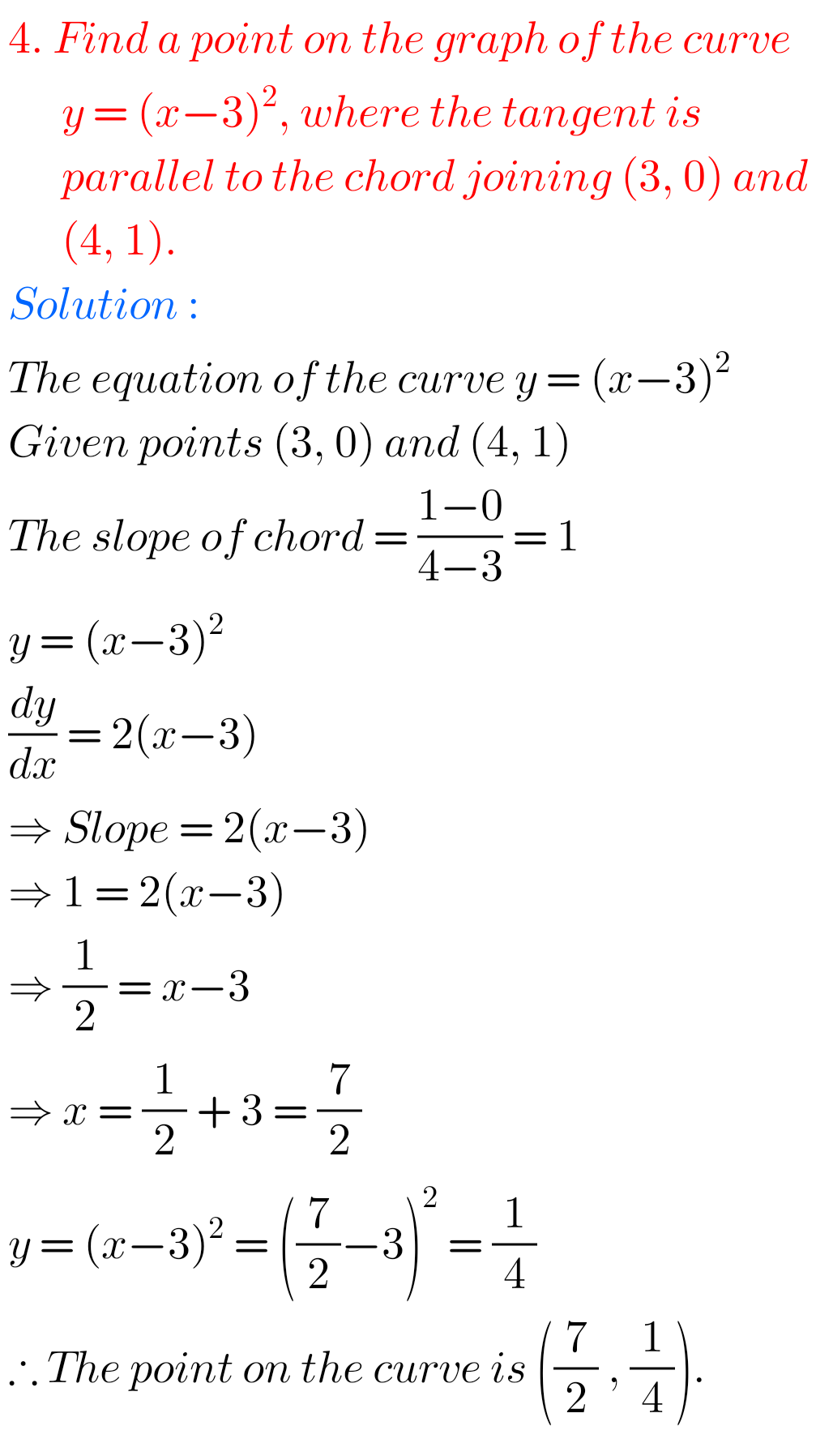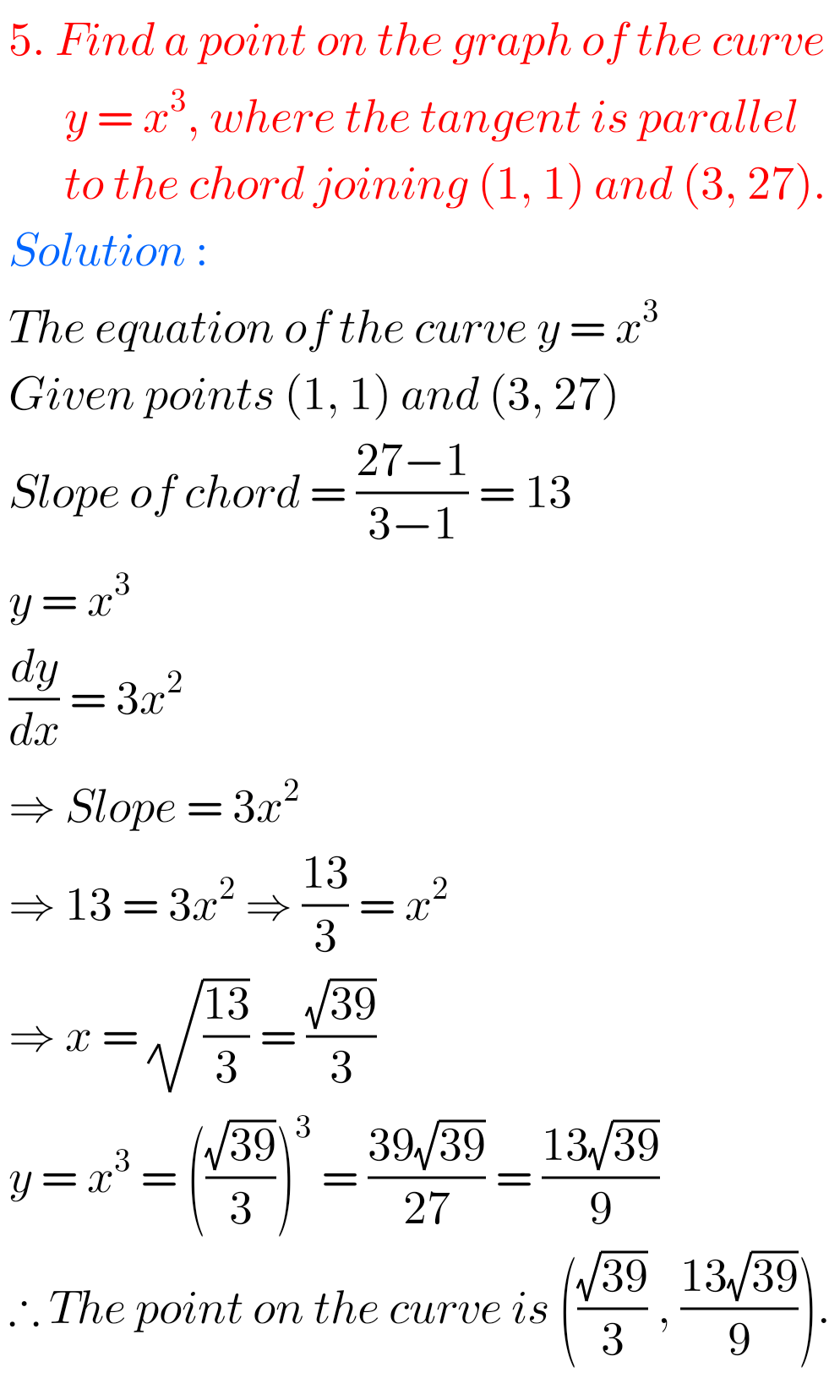## Inter 1st year maths 1B Applications of Derivatives solutions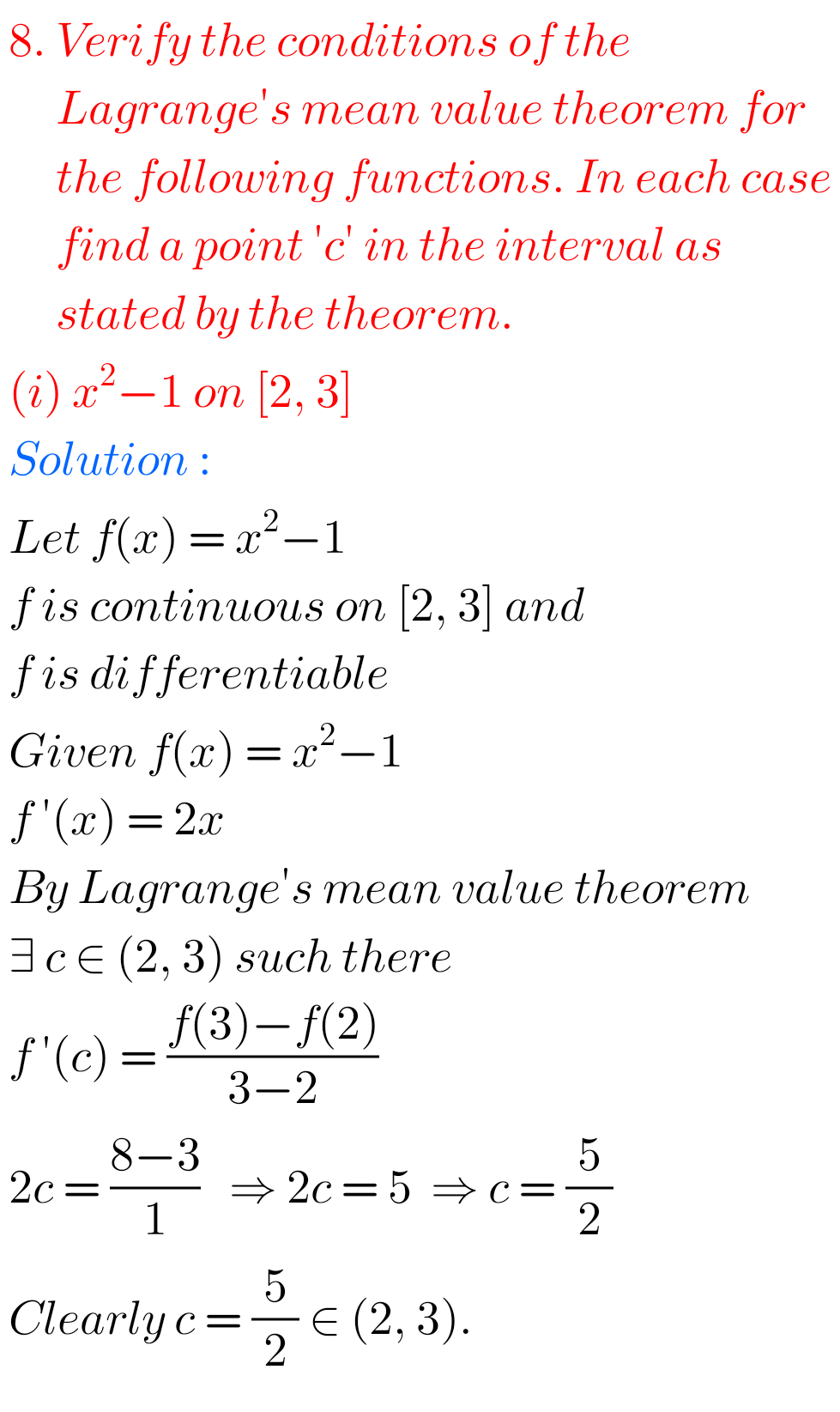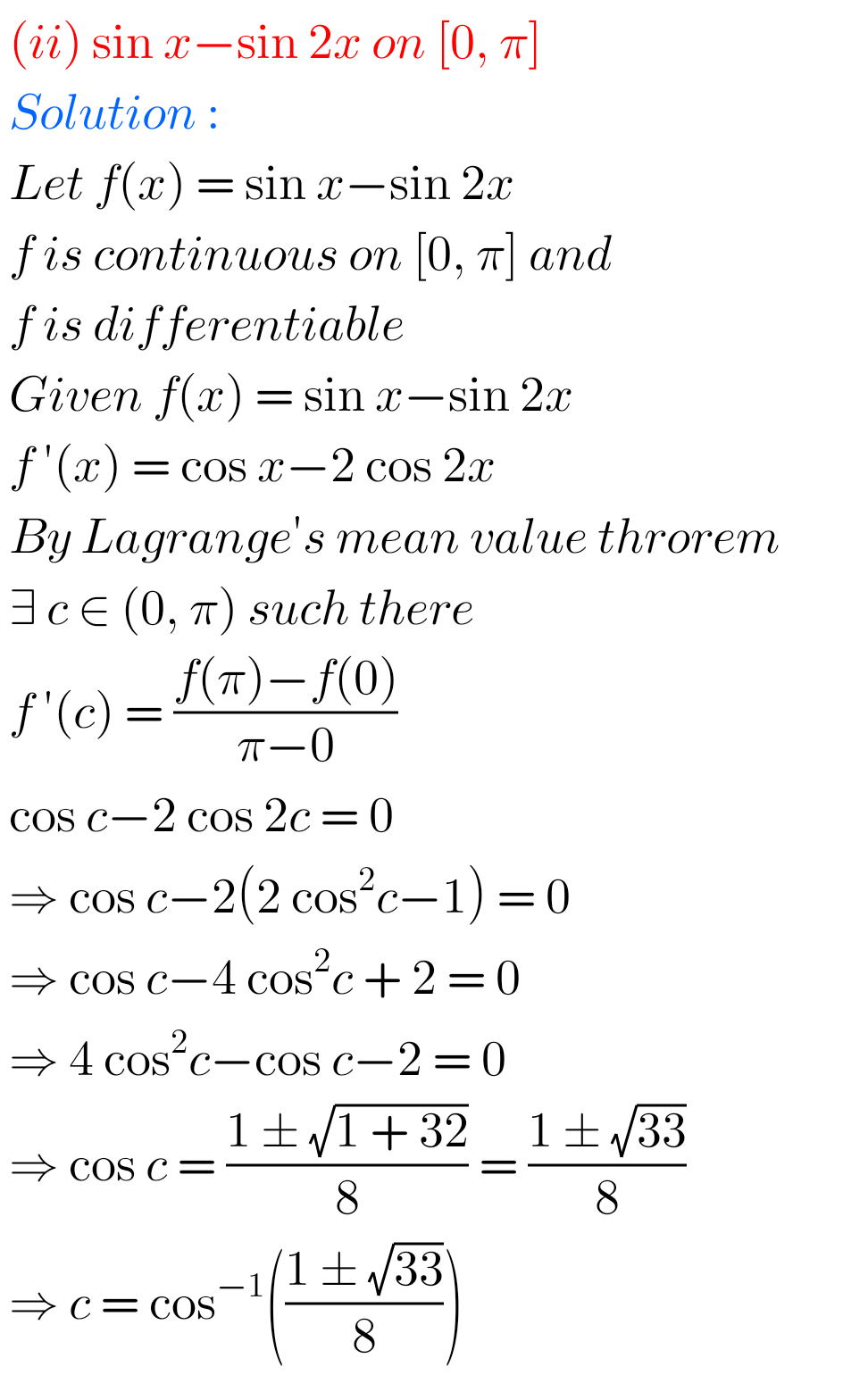Note : Observe the solutions and try them in your own method.SSC Maths Solutions class 10